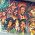## Wednesday, June 24, 2015

### 33 110 | 'Waited Their Turn to Be Shot' Headlines

Recall, the shooting was at 110 Calhoun St.  That number 110 is of great significance.
• Waited = 5+1+9+2+5+4 = 26
• their = 2+8+5+9+9 = 33
• turn = 2+3+9+5 = 19
• to = 2+6 = 8
• be = 2+5 = 7
• shot = 1+8+6+2 = 17/26
• Waited their turn to be shot = 110/119
Also notice the coding of 119 in addition to 110.  On the date of September 11, it was the 110 Story WTC buildings that crashed to the ground.
• Osama bin Laden = 110
• Adolf Hitler = 110 (Born on April 20, the 110th day of the year)
• Rockefeller = 110 (David Rockefeller proposed buildings, Nelson Rockefeller approved)
• President = 110
The man who made the remarks his William (Bill) Chumley.
• Bill = 2+9+3+3 = 17
• William = 5+9+3+3+9+1+4 = 34
• Chumley = 3+8+3+4+3+5+7 = 33
• Thirty-Three = 66/156
• South Carolina = 66/156

Interestingly enough, Bill Chumley's birthday comes during the time of the Pope's visit.
• 9/24/1947 = 9+24+19+47 = 99
• 9/24/1947 = 9+2+4+1+9+4+7 = 36
• 9/24/47 = 9+24+47 = 80 (Eighty = 5+9+7+8+20+25 = 74)
• 9/24 = 9+24 = 33

1.This may or may not have been pointed out already but, Dylann + Storm + Roof =209 (simple english gematria). July 28th is the 209th day of the year with  days remaining. 'Thirty three'=156

1.This comment has been removed by a blog administrator.

2.Peep This Zach, So supposely these two women who grandfather and father in law got killed in the Charleston Hoax started a campaign called "Hate wont win"
S.E.G=152, PT.G=53 BUT CHECK this out E.G=912
September 12 is the 255th day with 110 days left in year.
Hoax took place on 110 Calhun St..
Coincidence I think Not...SMDH

3.THAT is an interesting connection